# 检测和测量图像中的圆形目标

### 步骤 1：加载图像

1. 有不同颜色的塑料片，它们相对于背景有不同对比度。一方面，蓝色和红色塑料片在此背景上形成强烈的对比。另一方面，一些黄色塑料片与背景的对比不明显。

2. 请注意一些塑料片重叠在一起，而另一些塑料片则靠得很近，几乎互相接触。对于目标检测来说，场景中存在重叠的对象边缘和对象遮挡通常具有挑战性。

```rgb = imread('coloredChips.png'); imshow(rgb)```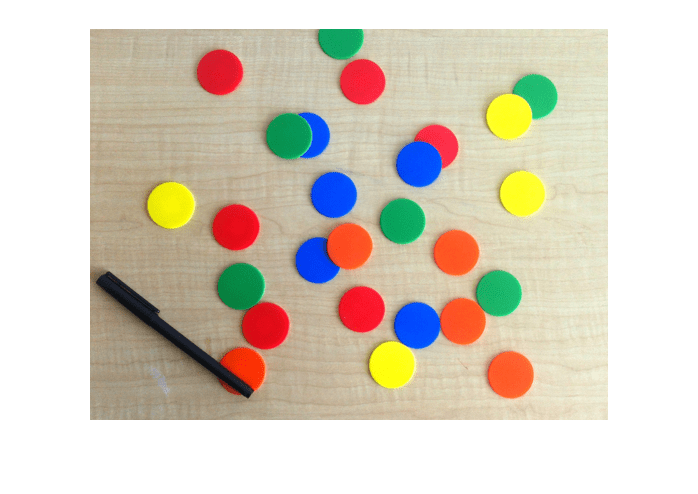### 步骤 2：确定搜索圆的半径范围

`d = drawline;`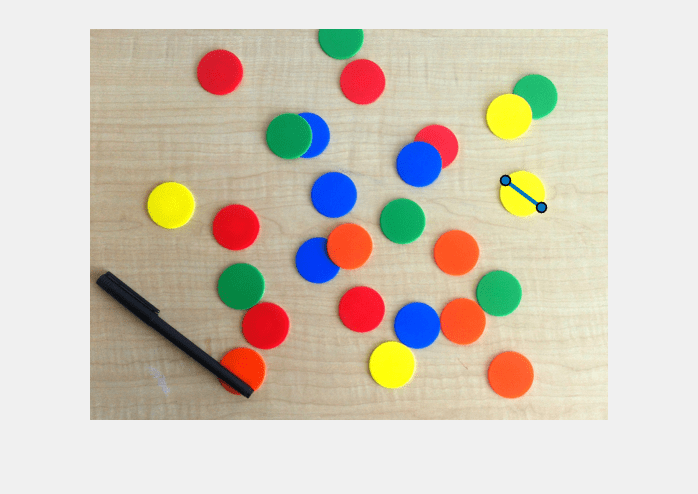```pos = d.Position; diffPos = diff(pos); diameter = hypot(diffPos(1),diffPos(2))```
```diameter = 45.3448 ```

### 步骤 3：寻找圆的初步尝试

`imfindcircles` 函数搜索符合半径范围的圆。搜索半径在 20 到 25 个像素范围内的圆。在此之前，最好要清楚对象是比背景亮还是比背景暗。要回答该问题，请看此图像的灰度版本。

```gray_image = rgb2gray(rgb); imshow(gray_image)```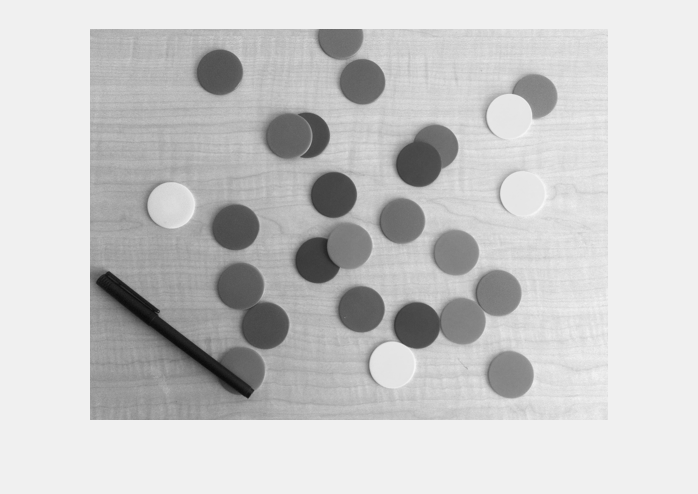`[centers,radii] = imfindcircles(rgb,[20 25],'ObjectPolarity','dark')`
```centers = [] radii = [] ```

### 步骤 4：提高检测敏感度

```[centers,radii] = imfindcircles(rgb,[20 25],'ObjectPolarity','dark', ... 'Sensitivity',0.9)```
```centers = 8×2 146.1895 198.5824 328.8132 135.5883 130.3134 43.8039 175.2698 297.0583 312.2831 192.3709 327.1316 297.0077 243.9893 166.4538 271.5873 280.8920 ```
```radii = 8×1 23.1604 22.5710 22.9576 23.7356 22.9551 22.9995 22.9055 23.0298 ```

### 步骤 5：在图像上绘制圆

```imshow(rgb) h = viscircles(centers,radii);```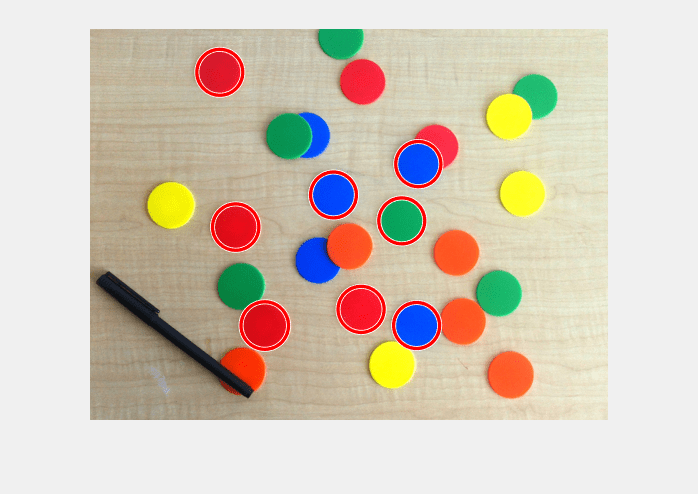```[centers,radii] = imfindcircles(rgb,[20 25],'ObjectPolarity','dark', ... 'Sensitivity',0.92); length(centers)```
```ans = 16 ```

```delete(h) % Delete previously drawn circles h = viscircles(centers,radii);```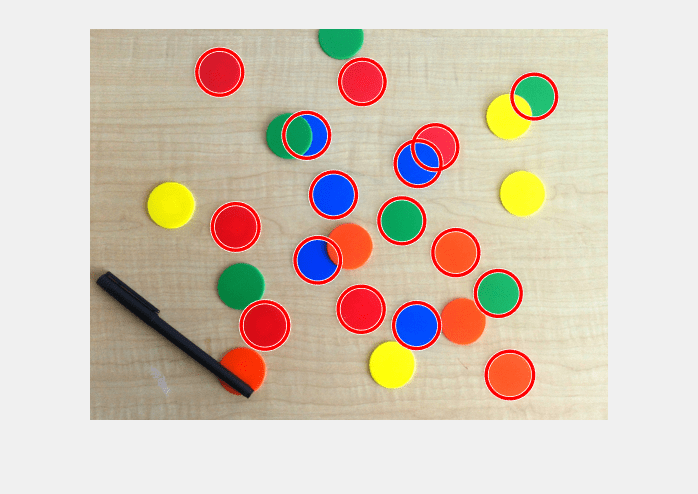### 步骤 6：使用第二种方法（两阶段）寻找圆

```[centers,radii] = imfindcircles(rgb,[20 25],'ObjectPolarity','dark', ... 'Sensitivity',0.92,'Method','twostage'); delete(h) h = viscircles(centers,radii);```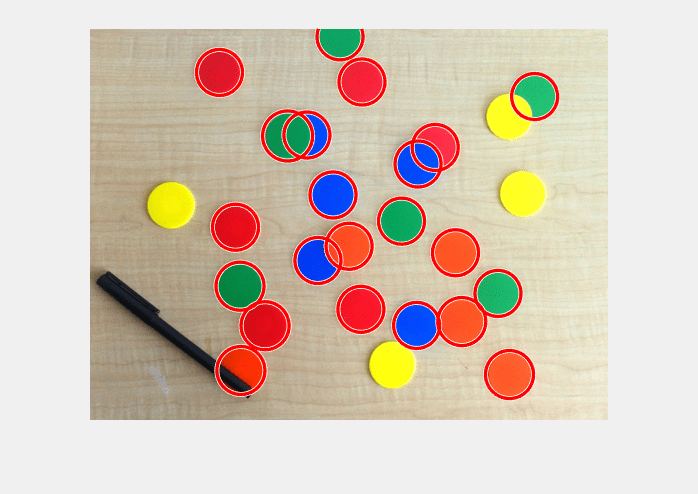```[centers,radii] = imfindcircles(rgb,[20 25],'ObjectPolarity','dark', ... 'Sensitivity',0.95); delete(h) viscircles(centers,radii);```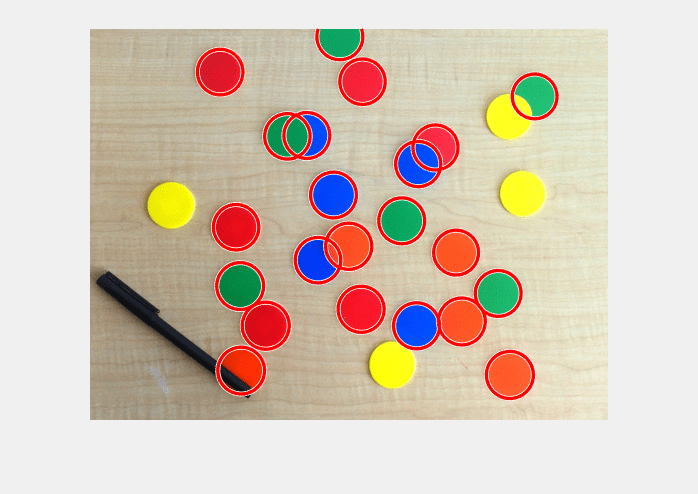### 步骤 7：为什么有些圆仍检测不到？

`imshow(gray_image)`### 步骤 8：在图像中找到“明亮”的圆

```[centersBright,radiiBright] = imfindcircles(rgb,[20 25], ... 'ObjectPolarity','bright','Sensitivity',0.92);```

### 步骤 9：用不同颜色绘制 'Bright' 圆

```imshow(rgb) hBright = viscircles(centersBright, radiiBright,'Color','b');```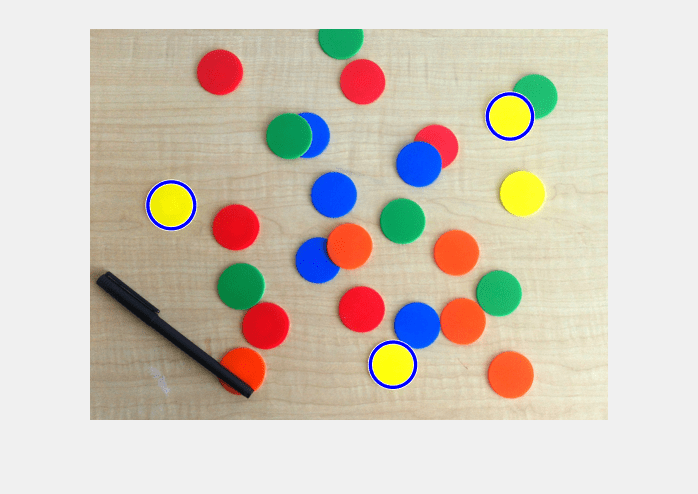### 步骤 10：降低 'EdgeThreshold' 的值

```[centersBright,radiiBright,metricBright] = imfindcircles(rgb,[20 25], ... 'ObjectPolarity','bright','Sensitivity',0.92,'EdgeThreshold',0.1); delete(hBright) hBright = viscircles(centersBright, radiiBright,'Color','b');```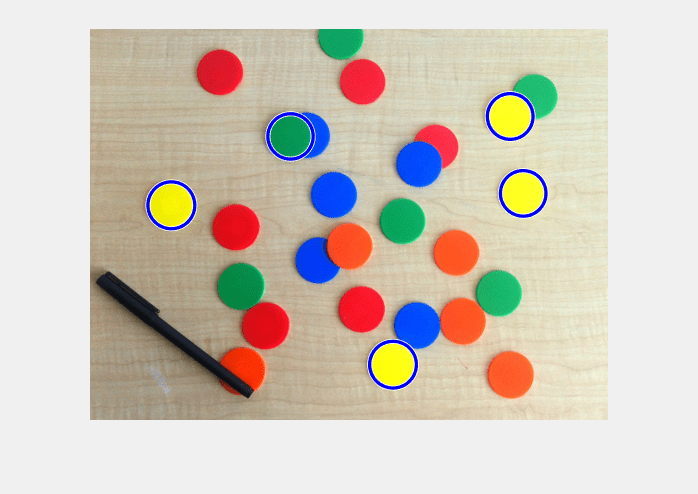### 步骤 11：同时绘制“暗”和“亮”圆

`h = viscircles(centers,radii);`